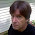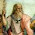## Sunday, July 11, 2010

### A Way From Perfection- Symmetry

Noether's Theorem

"For every continuous symmetry of the law of physics, there must exist a conservation law.

For every conservation law, there must exist a continuous symmetry"

### Conservation laws and symmetry

The symmetry properties of a physical system are intimately related to the conservation laws characterizing that system. Noether's theorem gives a precise description of this relation. The theorem states that each continuous symmetry of a physical system implies that some physical property of that system is conserved. Conversely, each conserved quantity has a corresponding symmetry. For example, the isometry of space gives rise to conservation of (linear) momentum, and isometry of time gives rise to conservation of energy.
The following table summarizes some fundamental symmetries and the associated conserved quantity.

 Class Invariance Conserved quantity Proper orthochronous Lorentz symmetry translation in time   (homogeneity) energy translation in space   (homogeneity) linear momentum rotation in space   (isotropy) angular momentum Discrete symmetry P, coordinate inversion spatial parity C, charge conjugation charge parity T, time reversal time parity CPT product of parities Internal symmetry (independent of spacetime coordinates) U(1) gauge transformation electric charge U(1) gauge transformation lepton generation number U(1) gauge transformation hypercharge U(1)Y gauge transformation weak hypercharge U(2) [U(1)xSU(2)] electroweak force SU(2) gauge transformation isospin SU(2)L gauge transformation weak isospin PxSU(2) G-parity SU(3) "winding number" baryon number SU(3) gauge transformation quark color SU(3) (approximate) quark flavor S((U2)xU(3)) [ U(1)xSU(2)xSU(3)] Standard Model

# Conservation law

In physics, a conservation law states that a particular measurable property of an isolated physical system does not change as the system evolves.

One particularly important physical result concerning conservation laws is Noether's Theorem, which states that there is a one-to-one correspondence between conservation laws and differentiable symmetries of physical systems. For example, the conservation of energy follows from the time-invariance of physical systems, and the fact that physical systems behave the same regardless of how they are oriented in space gives rise to the conservation of angular momentum.

A partial listing of conservation laws that are said to be exact laws, or more precisely have never been shown to be violated:
There are also approximate conservation laws. These are approximately true in particular situations, such as low speeds, short time scales, or certain interactions.

1.Nice! Your timing couldn't be better, as I am about to launch into a deep study of Group Representation Theory, and the promise all this stuff holds. I've already suggested the missing Symmetry is SU(4) Spin(6) for a T.O.E., but then along comes Martin Gleisner and tells us: there isn't a T.O.E.! Argh!

Emily Noether's story is somewhat sad, eh? Unable to obtain a Mathematics Lecturing job just because she was a woman, David Hilbert let her run one of his.

2.Hi Steven,

Long time no hear?:)

but then along comes Martin Gleisner and tells us: there isn't a T.O.E.! Argh!

In physics there is always that question mark about unification at "some point," so the idea is still out there, it just does not ring a bell for some to ponder.

Laplace: "An intellect which at a certain moment would know all forces that set nature in motion, and all positions of all items of which nature is composed, if this intellect were also vast enough to submit these data to analysis, it would embrace in a single formula the movements of the greatest bodies of the universe and those of the tiniest atom; for such an intellect nothing would be uncertain and the future just like the past would be present before its eyes.
— Essai philosophique sur les probabilités, Introduction. 1814

So the idea of a TOE has been around for sometime. See current post entry for today.

As to Emmy Noether's story now in full light of our day and age, yes, it is a remnant of an attitude of men that should have long been exterminated from our societies.

Best,